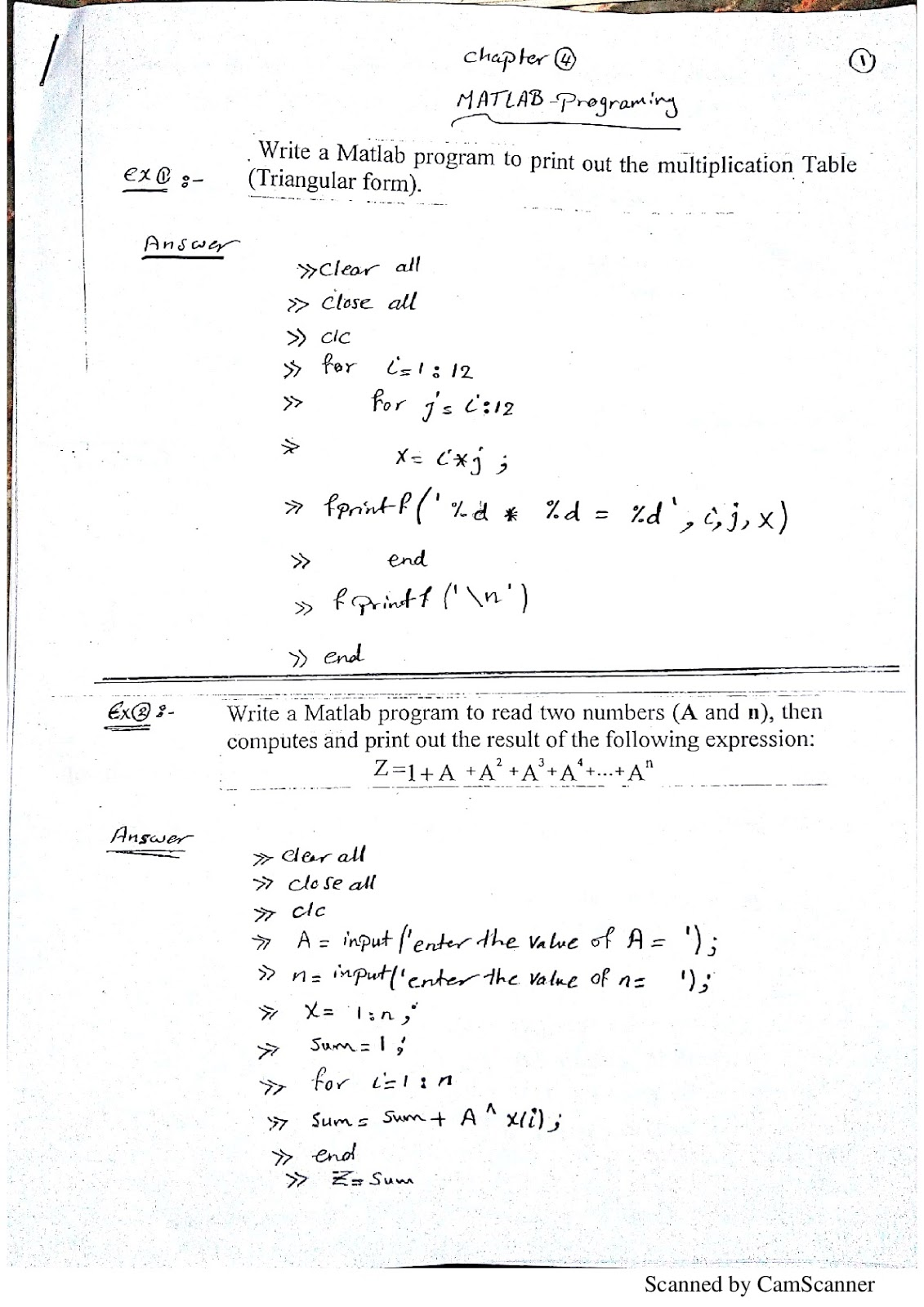# Matlab Programming revision-examples and problems with their model answer in editor and command window

####Ex 1: Write a matlab program to print out the multiplication table (Triangular form)

مثال 1 :كتابة برنامج matlab لطباعة جدول الضرب
clear all
close all
clc

for a=1:12
for b=a:12
x=a*b
fprintf('%d*%d = %d',a,b,x)
end
fprintf('\n')
end

x =1       1*1 = 1
x =2       1*2 = 2
x =3       1*3 = 3
x =4       1*4 = 4
x =5       1*5 = 5
x =6       1*6 = 6
x =7       1*7 = 7
x =8       1*8 = 8
x =9       1*9 = 9
x =10     1*10 = 10
x =11     1*11 = 11
x =12     1*12 = 12
x =4       2*2 = 4
x =6       2*3 = 6
x =8       2*4 = 8
x =10     2*5 = 10
x =12     2*6 = 12
x =14     2*7 = 14
x =16     2*8 = 16
x =18     2*9 = 18
x =20     2*10 = 20
x =22     2*11 = 22
x =24     2*12 = 24
x =9       3*3 = 9
x =12     3*4 = 12
x =15     3*5 = 15
x =18     3*6 = 18
x =21     3*7 = 21
x =24     3*8 = 24
x =27     3*9 = 27
x =30     3*10 = 30
x =33     3*11 = 33
x =36     3*12 = 36
x =16     4*4 = 16
x =20     4*5 = 20
x =24     4*6 = 24
x =28     4*7 = 28
x =32     4*8 = 32
x =36     4*9 = 36
x =40     4*10 = 40
x =44     4*11 = 44
x =48     4*12 = 48
x =25     5*5 = 25
x =30     5*6 = 30
x =35     5*7 = 35
x =40     5*8 = 40
x =45     5*9 = 45
x =50     5*10 = 50
x =55     5*11 = 55
x =60     5*12 = 60
x =36     6*6 = 36
x =42     6*7 = 42
x =48     6*8 = 48
x =54     6*9 = 54
x =60     6*10 = 60
x =66     6*11 = 66
x =72     6*12 = 72
x =49     7*7 = 49
x =56     7*8 = 56
x =63     7*9 = 63
x =70     7*10 = 70
x =77     7*11 = 77
x =84     7*12 = 84
x =64     8*8 = 64
x =72     8*9 = 72
x =80     8*10 = 80
x =88     8*11 = 88
x =96     8*12 = 96
x =81     9*9 = 81
x =90     9*10 = 90
x =99     9*11 = 99
x =108   9*12 = 108
x =100   10*10 = 100
x =110   10*11 = 110
x =120   10*12 = 120
x =121   11*11 = 121
x =132   11*12 = 132
x =144   12*12 = 144

#### Ex 2: Write a matlab program to read two number (A and n),then computes and print out the result of the following expression :Z=1+A+A^2+A^3+A^4+...+A^n

clear all
close all
clc
A=input('enter the value of A= ')
N=input('enter the value of N= ')
x=1:N
sum=1
for b=1:N
sum=sum+A^x(b)
end
z=sum
enter the value of A= 3
A =
3
enter the value of N= 3
N =
3
x =
1     2     3
sum =
1
sum =
4
sum =
13
sum =
40
z =
40

####Ex 3:Write a Matlab program to compute the summation of all even numbers from 0 to 100.

مثال3 :اكتب برنامج باستخدام لغة البرمجة ماتلاب لحساب الاعداد الزوجية  من 1:100

#### Ex 4:Write a Matlab program to compute the summation of all odd numbers from 0 to 100.

مثال4 :اكتب برنامج ماتلاب لحساب الاعداد الفردية من 1:100

#### Ex 5:Write a Matlap program to calculate the factorial of any number(n!)

مثال5 :اكتب برنامج ماتلاب لحساب مضروب اي عدد

#### clear all close all clc d1=input('Enter the present day         ='); m1=input('Enter the present month   ='); y1=input('Enter the present year        ='); d2=input('Enter the day of birth         ='); m2=input('Enter the month of birth   ='); y2=input('Enter the year of birth        ='); day=d1-d2; if day<0 0="" br="">

<0 br="" style="font-weight: normal;">    day=day+30
m1=m1-1
end
month=m1-m2;
if month<0 0="">
<0 br=""><0 br="" style="font-weight: normal;">    month=month+12
y1=y1-1
end
year=y1-y2;
x=[day month year];
disp('    Day   Month  Year')
disp(x)
Enter the present day     =28
Enter the present month =5
Enter the present year    =2018
Enter the day of birth     =14
Enter the month of birth=4
Enter the year of birth   =2001
Day   Month  Year

14       1          17

حجم الخط
+
16
-
تباعد السطور
+
2
-